# PSAT Math : How to find the surface area of a tetrahedron

## Example Questions

### Example Question #83 : Solid Geometry

A regular tetrahedron has four congruent faces, each of which is an equilateral triangle.

A given tetrahedron has edges of length six inches. Give the total surface area of the tetrahedron.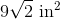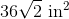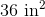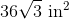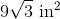Explanation:

The area of an equilateral triangle is given by the formula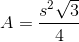Since there are four equilateral triangles that comprise the surface of the tetrahedron, the total surface area is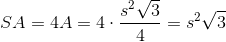Substitute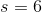: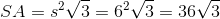square inches.

### Example Question #84 : Solid Geometry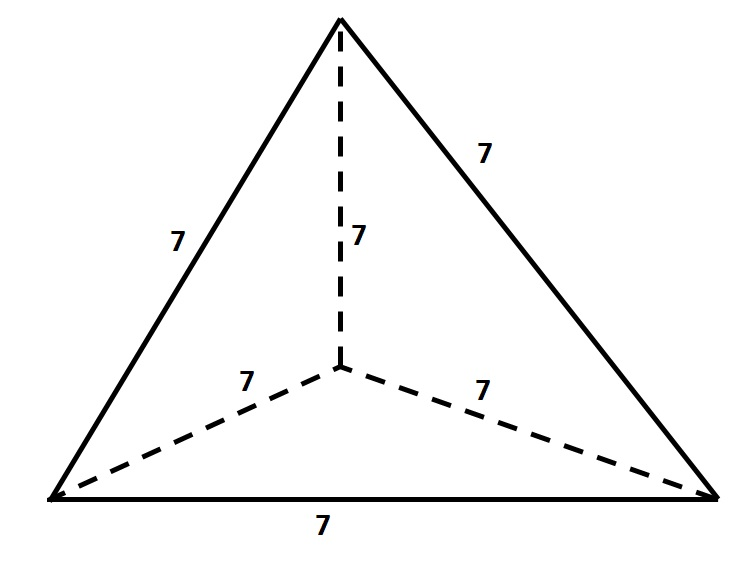Give the surface area of the above tetrahedron, or four-faced solid, to the nearest tenth.

Insufficient information is given to answer the question.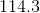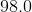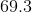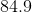Explanation:

The tetrahedron has four faces, each of which is an equilateral triangle with sidelength 7. Each face has area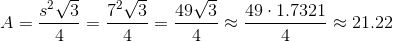The total surface area is four times this, or about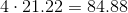.

Rounded, this is 84.9.